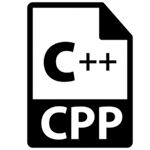# C++函数-通过值调用和引用调用

## 在C++中通过值调用

```#include
using namespace std;
void change(int data);
int main()
{
int data = 3;
change(data);
cout << "Value of the data is: " << data<< endl;
return 0;
}
void change(int data)
{
data = 5;
}
```

```Value of the data is: 3
```

## 在C++中通过引用调用

```#include
using namespace std;
void swap(int *x, int *y)
{
int swap;
swap=*x;
*x=*y;
*y=swap;
}
int main()
{
int x=500, y=100;
swap(&x, &y);  // passing value to function
cout<<"Value of x is: "<

执行上面代码，得到以下结果 -
Value of x is: 100
Value of y is: 500

在C++中通过值调用和通过引用调用的区别

序号

通过值调用

通过引用调用

1

将值的副本传递给函数

将值的地址传递给函数

2

在函数内部进行的更改不会反映在函数外部

在函数内部进行的更改也反映在函数外部

3

实际和形式参数将在不同的内存位置创建

实际和形式参数将在同一内存位置创建

本站文章除注明转载外，均为本站原创或编译
欢迎任何形式的转载，但请务必注明出处，尊重他人劳动共创优秀实例教程
转载请注明：文章转载自：代码驿站 [http:/www.codeinn.net]
本文标题：C++函数-通过值调用和引用调用
本文地址：http://www.codeinn.net/cplus/1763.html

```